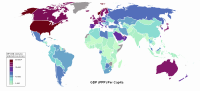# Computational economics

﻿
Computational economics
General categories EconomicsEconomies by region Microeconomics · Macroeconomics History of economic thought Methodology · Mainstream & heterodox Mathematical economics Game theory  · Optimization Computational · Econometrics Experimental · National accounting Lists Economy: concept and history Business and Economics Portal

Computational economics is a research discipline at the interface between computer science and economic and management science. Areas encompassed include agent-based computational modeling, computational modeling of dynamic macroeconomic systems and transaction costs, other applications in mathematical economics, computational econometrics and statistics, computational finance, computational tools for the design of automated Internet markets, programming tools specifically designed for computational economics, and pedagogical tools for the teaching of computational economics. Some of these areas are unique to computational economics, while others extend traditional areas of economics by solving problems that are difficult to study without the use of computers. 

Computational economics researchers use computational tools both for computational economic modeling and for the computational solution of analytically and statistically formulated economic problems. An important example is agent-based computational economics (ACE) is the computational study of economic processes modeled as dynamic systems of interacting agents. Here "agent" refers broadly to a bundle of data and behavioral methods representing an entity constituting part of a computationally constructed world. Agents can represent social, biological, and/or physical entities. Starting from initial conditions determined by the modeler, an ACE model develops forward through time driven solely by agent interactions.

Computational solution tools include for example software for carrying out various matrix operations (e.g. matrix inversion) and for solving systems of linear and nonlinear equations. For a repository of public-domain computational solution tools, visit here.

The following journals specialize in computational economics: Computational Economics, Journal of Applied Econometrics,Journal of Economic Dynamics and Control, and the Journal of Economic Interaction and Coordination.

Wikimedia Foundation. 2010.

### Look at other dictionaries:

• Agent-Based Computational Economics — (ACE) is the computational study of economic processes modeled as dynamic systems of interacting agents.OverviewThe agents in ACE models can represent individuals (e.g. people), social groupings (e.g. firms), biological entities (e.g. growing… …   Wikipedia

• Computational — may refer to: Computer Computational algebra Computational Aeroacoustics Computational and Information Systems Laboratory Computational and Systems Neuroscience Computational archaeology Computational auditory scene analysis Computational biology …   Wikipedia

• Computational intelligence — (CI) is a set of Nature inspired computational methodologies and approaches to address complex problems of the real world applications to which traditional (first principles, probabilistic, black box, etc.) methodologies and approaches are… …   Wikipedia

• Computational science — Not to be confused with computer science …   Wikipedia

• Economics of global warming — This article describes the economics of global warming and climate change. Contents 1 Definitions 2 Climate change science 3 Scenarios 4 Trends and projections …   Wikipedia

• Computational finance — Computational finance, also called financial engineering, is a cross disciplinary field which relies on computational intelligence, mathematical finance, numerical methods and computer simulations to make trading, hedging and investment decisions …   Wikipedia

• Computational elasticity — In economics, elasticity is the ratio of the percent change in one variable to the percent change in another variable. Computational elasticity is the application of this concept to how computer systems scale with relation to temporal monetary… …   Wikipedia

• Computational sociology — Sociology …   Wikipedia

• Computational informatics — Informatics is the academic field concerned with the study of the foundations, theory, structure, communication, and use of information. Computational informatics, as a subfield of informatics, studies information using computational… …   Wikipedia